# matplotlib常见函数- plt.rcParams、matshow/坐标轴设置

28 篇文章 13 订阅

## 1、plt.rcParams

plt（matplotlib.pyplot）使用rc配置文件来自定义图形的各种默认属性，称之为“rc配置”或“rc参数”。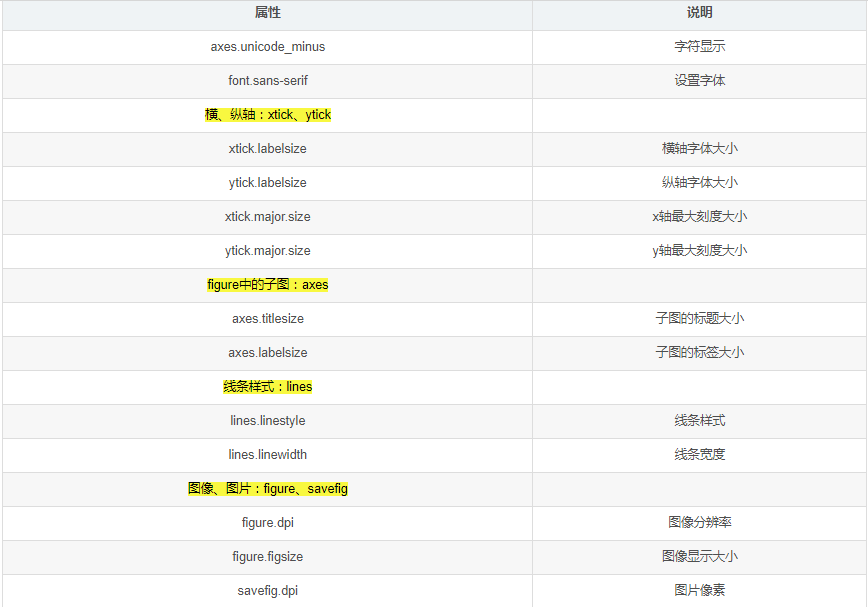import numpy as np
import matplotlib.pyplot as plt
###%matplotlib inline    #jupyter可以用，这样就不用plt.show()

#生成数据
x = np.linspace(0, 4*np.pi)
y = np.sin(x)
#设置rc参数显示中文标题
#设置字体为SimHei显示中文
plt.rcParams['font.sans-serif'] = 'SimHei'
#设置正常显示字符
plt.rcParams['axes.unicode_minus'] = False
plt.title('sin曲线')
#设置线条样式
plt.rcParams['lines.linestyle'] = '-.'
#设置线条宽度
plt.rcParams['lines.linewidth'] = 3
#绘制sin曲线
plt.plot(x, y, label='$sin(x)$')

plt.savefig('sin.png')
plt.show()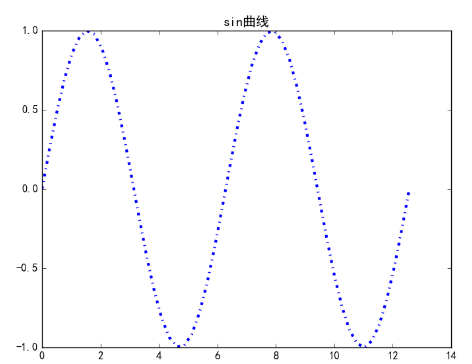plt.rcParams['savefig.dpi'] = 300 #图片像素
plt.rcParams['figure.dpi'] = 300 #分辨率
plt.savefig(‘plot123_2.png’, dpi=200)#指定分辨率
# 默认的像素：[6.0,4.0]，分辨率为100，图片尺寸为 600&400
# 指定dpi=200，图片尺寸为 1200*800
# 指定dpi=300，图片尺寸为 1800*1200

plt.rcParams['figure.figsize'] = (8.0, 4.0)        # 图像显示大小
plt.rcParams['image.interpolation'] = 'nearest'  # 最近邻差值: 像素为正方形
#Interpolation/resampling即插值，是一种图像处理方法，它可以为数码图像增加或减少象素的数目。

plt.rcParams['image.cmap'] = 'gray'  # 使用灰度输出而不是彩色输出

plt.axis('off')    #打印图片的时候不显示坐标轴

## 2、matshow函数

A是绘制的矩阵，一个矩阵元素对应一个图像像素。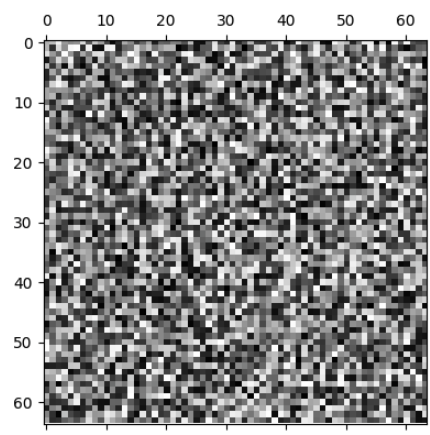plt.plot(A, "r-+", linewidth=2, label="train")
plt.plot(B, "b-", linewidth=3, label="val")
plt.legend(loc="upper right", fontsize=14)   # 设置位置
plt.xlabel("Training set size", fontsize=14) # 标签
plt.ylabel("RMSE", fontsize=14)
plt.axis([0, 80, 0, 3])#表示要显示图形的范围
plt.xticks(np.arange(0, 81, step=20))#设置刻度
plt.yticks(np.arange(0, 4, step=1))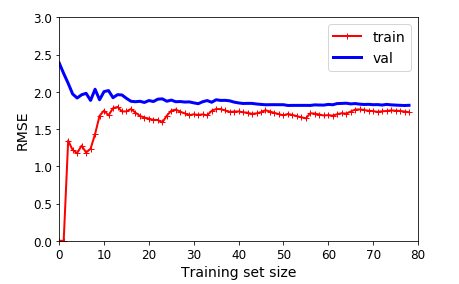## Axes - Subplot - Axis 之间到底是个什么关系

fig = plt.figure()
plt.show()

ax2 = fig.add_subplot(212)

用画板和画纸来做比喻的话，figure就好像是画板，是画纸的载体， 但是具体画画等操作是在画纸上完成的。 在pyplot中，画纸的概念对应的就是Axes/Subplot。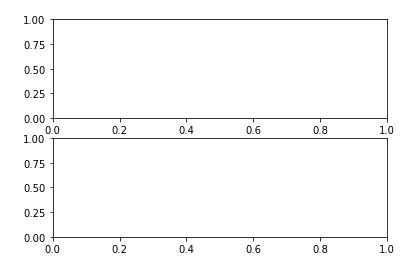### 对比:

figure (1) VS figure()
figure()操作就是创建或者调用画图板，缺省情况下系统会创建figure(1)作为画图板。使用时遵循就近原则，所有画图操作是在最近一次调用的画图板上实现。

axes() VS subplot()
pyplot.axes([x, y, w, h])是用来在画图板上确认图形区的位置和大小的函数，x,y表示图形区左下角相对于画图板的坐标，w,h表示图形区的宽高。（缺省时该操作在figure(1)上操作）

pyplot.subplot(abc)本质也是用来确认图形区在画图板上位置大小的函数，区别是该函数将画图板按a行b列等分，然后逐行编号，并选择编号为c的区域作为图形区用来绘图。这是一个axes()操作的高级封装，方便用户使用。subplot(233)表示2行3列的第3个位置（即，第1行第三个区域）

axes() VS axis()
axes([x, y, w, h])用来设定图形区;

axis([x_left, x_right, y_bottom, y_top])是用来设置所绘制图形的视窗大小的，表示直接展示的图形是需要满足参数中范围的值，直观表现是绘图区实际展示的坐标范围。

subplot() VS plot()
subplot用来生成图形区;

plot是实际使用的绘图函数，类似的函数还有hist等，plot操作遵守就近原则，即作用在最近一次使用的图形区上。01-301371
03-106万+
09-1336
03-032万+
10-094万+
02-095457
01-271万+
03-052万+
10-052590
01-03
12-09
01-27410
09-204万+
02-062550点击重新获取扫码支付余额充值